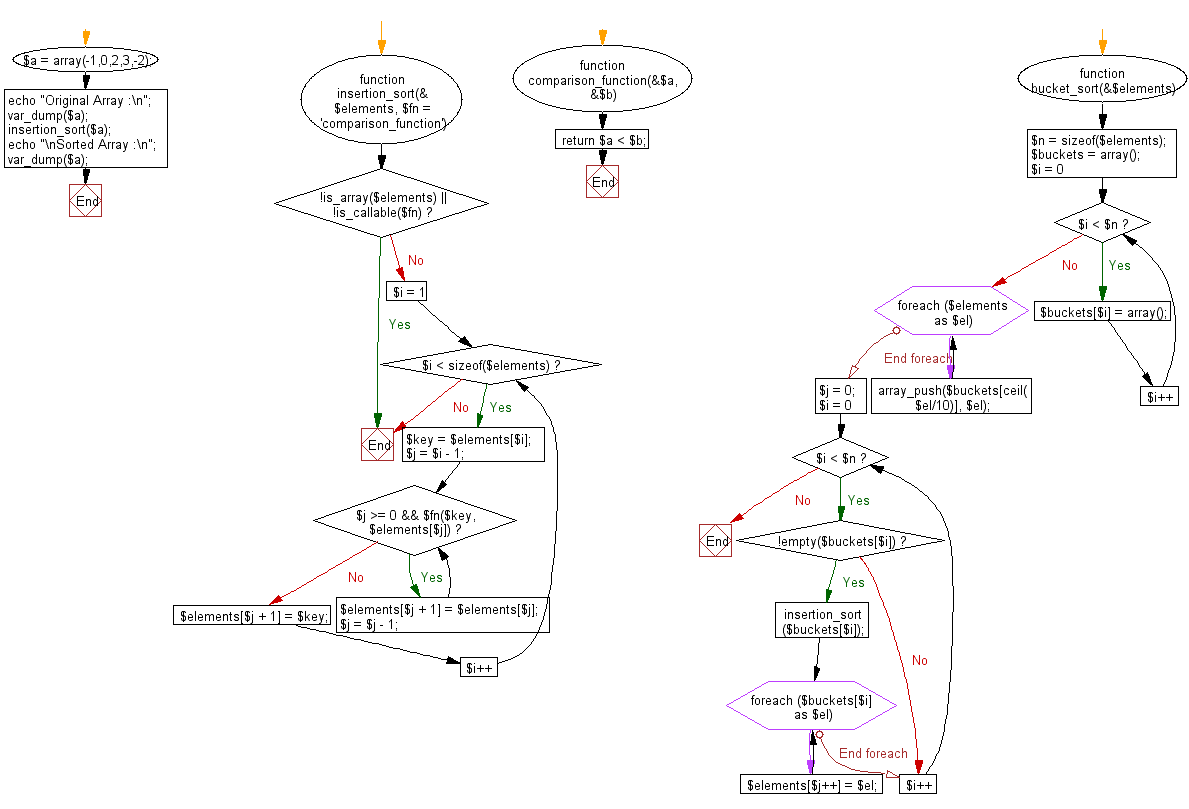﻿ PHP: Sort a list of elements using Bucket sort - w3resource

# PHP Searching and Sorting Algorithm: Bucket sort

## PHP Searching and Sorting Algorithm: Exercise-10 with Solution

Write a PHP program to sort a list of elements using Bucket sort.

Sample Solution:

PHP Code:

``````<?php
function insertion_sort(&\$elements, \$fn = 'comparison_function') {
if (!is_array(\$elements) || !is_callable(\$fn)) return;
for (\$i = 1; \$i < sizeof(\$elements); \$i++) {
\$key = \$elements[\$i]; // This will be \$a in comparison function.
// \$key will be the right side element that will be
// compared against the left sorted elements. For
// min to max sort, \$key should be higher than
// \$elements to \$elements[\$j]

\$j = \$i - 1; // this will be in \$b in comparison function
while ( \$j >= 0 && \$fn(\$key, \$elements[\$j]) ) {
\$elements[\$j + 1] = \$elements[\$j]; // shift right
\$j = \$j - 1;
}
\$elements[\$j + 1] = \$key;
}
}
/**
* Comparison function used to compare each element.
* @param mixed \$a
* @param mixed \$b
* @returns bool True iff \$a is less than \$b.
*/
function comparison_function(&\$a, &\$b) {
return \$a < \$b;
}

function bucket_sort(&\$elements) {
\$n = sizeof(\$elements);
\$buckets = array();
// Initialize the buckets.
for (\$i = 0; \$i < \$n; \$i++) {
\$buckets[\$i] = array();
}
// Put each element into matched bucket.
foreach (\$elements as \$el) {
array_push(\$buckets[ceil(\$el/10)], \$el);
}
// Sort elements in each bucket using insertion sort.
\$j = 0;
for (\$i = 0; \$i < \$n; \$i++) {
// sort only non-empty bucket
if (!empty(\$buckets[\$i])) {
insertion_sort(\$buckets[\$i]);
// Move sorted elements in the bucket into original array.
foreach (\$buckets[\$i] as \$el) {
\$elements[\$j++] = \$el;
}
}
}
}
\$a = array(-1,0,2,3,-2);
echo "Original Array :\n";
insertion_sort(\$a); // Sort the elements
echo "\nSorted Array :\n";
var_dump(\$a);
?>
```
```

Sample Output:

```Original Array :
array(5) {
=>
int(-1)
=>
int(0)
=>
int(2)
=>
int(3)
=>
int(-2)
}

Sorted Array :
array(5) {
=>
int(-2)
=>
int(-1)
=>
int(0)
=>
int(2)
=>
int(3)
}
```

Flowchart :PHP Code Editor:

Have another way to solve this solution? Contribute your code (and comments) through Disqus.

What is the difficulty level of this exercise?

Test your Programming skills with w3resource's quiz.

﻿